The major aspects of lipid metabolism are involved with Fatty Acid Oxidation to produce energy or the synthesis of lipids which is called Lipogenesis.
Lipid metabolism is closely connected to the metabolism of carbohydrates which may be converted to fats. This can be seen in the diagram on the left. The metabolism of both is upset by diabetes mellitus.
The first step in lipid metabolism is the hydrolysis of the lipid in the cytoplasm to produce glycerol and fatty acids. Since glycerol is a three carbon alcohol, it is metabolized quite readily into an intermediate in glycolysis, dihydroxyacetone phosphate. The last reaction is readily reversible if glycerol is needed for the synthesis of a lipid.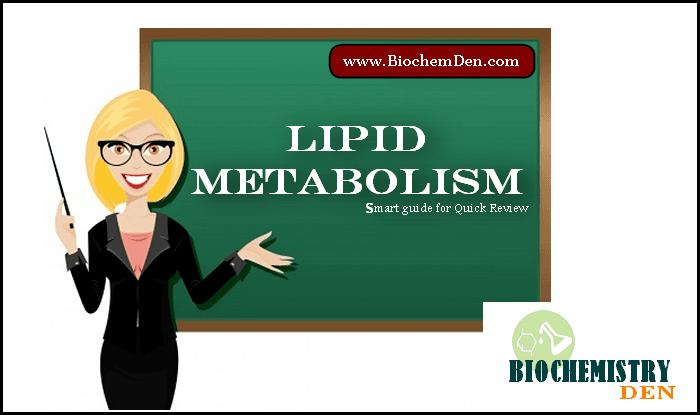The hydroxyacetone, obtained from glycerol is metabolized into one of two possible compounds. Dihydroxyacetone may be converted into pyruvic acid through the glycolysis pathway to make energy. In addition, the dihydroxyacetone may also be used in gluconeogenesis to make glucose-6-phosphate for glucose to the blood or glycogen depending upon what is required at that time.
Fatty acids are oxidized to acetyl CoA in the mitochondria using the fatty acid spiral. The acetyl CoA is then ultimately converted into ATP, CO2, and H2O using the citric acid cycle and the electron transport chain.
Fatty acids are synthesized from carbohydrates and occasionally from proteins. Actually, the carbohydrates and proteins have first been catabolized into acetyl CoA. Depending upon the energy requirements, the acetyl CoA enters the citric acid cycle or is used to synthesize fatty acids in a process known as LIPOGENESIS.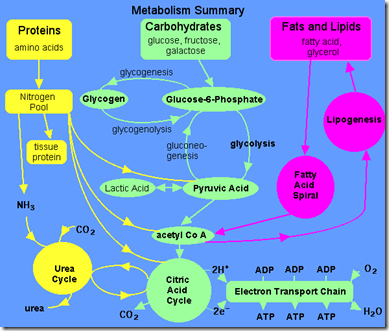## Energy Production Fatty Acid Oxidation:

### “Visible” ATP:

In the fatty acid spiral, there is only one reaction which directly uses ATP and that is in the initiating step. So this is a loss of ATP and must be subtracted later. A large amount of energy is released and restored as ATP during the oxidation of fatty acids. The ATP is formed from both the fatty acid spiral and the citric acid cycle.

### Connections to Electron Transport and ATP:

One turn of the fatty acid spiral produces ATP from the interaction of the coenzymes FAD (step 1) and NAD+ (step 3) with the electron transport chain. Total ATP per turn of the fatty acid spiral is:
Electron Transport Diagram – (e.t.c.)
Step 1 – FAD into e.t.c. = 2 ATP
Step 3 – NAD+ into e.t.c. = 3 ATP
————————————
Total ATP per turn of spiral = 5 ATP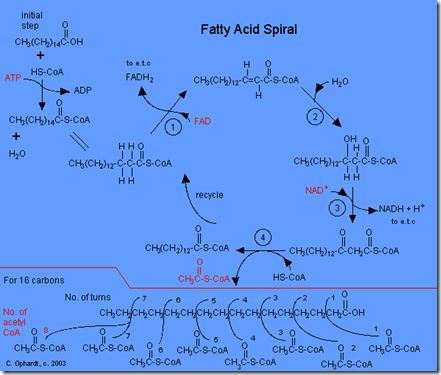In order to calculate total ATP from the fatty acid spiral, you must calculate the number of turns that the spiral makes. Remember that the number of turns is found by subtracting one from the number of acetyl CoA produced. See the graphic on the left bottom.
Example with Palmitic Acid = 16 carbons = 8 acetyl groups
Number of turns of fatty acid spiral = 8-1 = 7 turns
ATP from fatty acid spiral = 7 turns and 5 per turn = 35 ATP.
This would be a good time to remember that single ATP that was needed to get the fatty acid spiral started. Therefore subtract it now.
NET ATP from Fatty Acid Spiral = 35 – 1 = 34 ATP

## Review ATP Summary for Citric Acid Cycle:

The acetyl CoA produced from the fatty acid spiral enters the citric acid cycle. When calculating ATP production, you have to show how many acetyl CoA are produced from a given fatty acid as this controls how many “turns” the citric acid cycle makes.
Starting with acetyl CoA, how many ATP are made using the citric acid cycle? E.T.C = electron transport chain.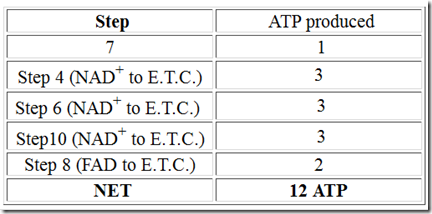###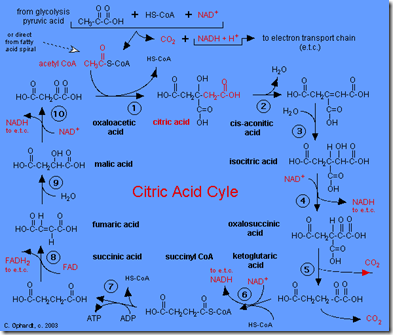ATP Summary for Palmitic Acid – Complete Metabolism:

The phrase “complete metabolism” means do reactions until you end up with carbon dioxide and water. This also means to use fatty acid spiral, citric acid cycle, and electron transport as needed.
Starting with palmitic acid (16 carbons) how many ATP are made using fatty acid spiral? This is a review of the above panel E.T.C = electron transport chain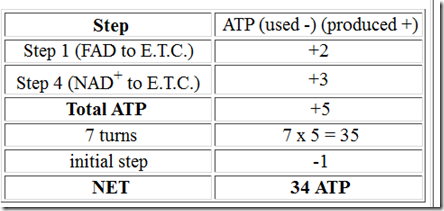The fatty acid spiral ends with the production of 8 acetyl CoA from the 16 carbon palmitic acid. Starting with one acetyl CoA, how many ATP are made using the citric acid cycle? Above panel gave the answer of 12 ATP per acetyl CoA.
E.T.C = electron transport chain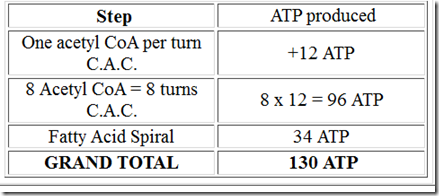[mtouchquiz 19]